# WebMO Help - Symmetry

## Molecular Symmetry

Molecular symmetry can be used to understand bonding in molecules, predict infrared and raman active transitions, and simplify quantum chemistry calculations. The symmetry of a molecule is described by a molecular point group, which is determined by the presence of symmetry elements (inversion points, proper and improper axes, and mirror planes). WebMO can detect and draw symmetry elements, as well as calculate the point group of a molecule.

Since computer calculations include numerical tolerances, many times a molecular structure is "nearly symmetric." WebMO can identity the closest point group and then symmetrize the molecule.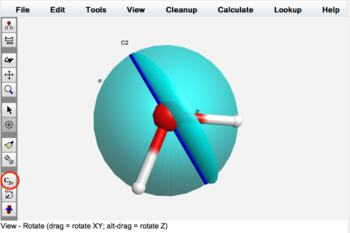Water (C2v)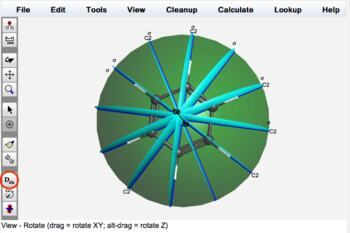Benzene (D6h)

## Symmetry Examples

An molecule from each point group is provided in the Fragment Library. From the Build tool, choose Build: Fragment... to open the Choose Fragment dialog box. For Category, choose Symmetry Examples. For Fragment, choose the desired point group example. Click OK. Click in the 3-D editor to insert the entire fragment. The Symmetrize icon should indicate the point group. Optionally click the Display Symmetry Elements icon (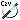) to display the various symmetry elements.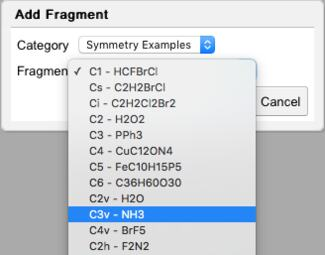Fragment Library with Symmetry Examples

## Displaying Symmetry Elements

Symmetry elements for the detected point group can be displayed by clicking the Display Symmetry Elements icon () in the 3-D Editor or Visualizer toolbar. Initially all symmetry elements are selected for display.

Selected symmetry elements can be displayed or hidden using the Calculate: Symmetry: Select Symmetry Elements... menu item. Clicking the Display Symmetry Elements icon toggles the display of selected symmetry elements.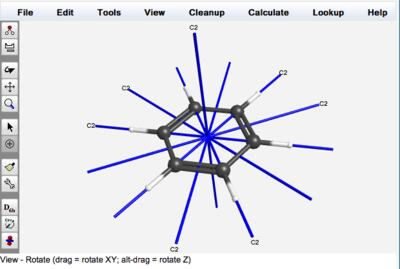Displaying C2 Axes of Benzene

## Detecting Symmetry

The point group of the current structure is automatically determined and displayed in the toolbar of the 3-D editor. If the molecule is exactly symmetry, the point group is display in black, e.g., C2v. If the molecule is nearly symmetry, the point group is displayed in red with an asterisk, e.g., C2v*.

The symmetry of the current structure can also be detected using the Calculate: Symmetry: Symmetrize Molecule... menu item. Clicking Detect Symmetry will report both the exact point group of the molecule and the approximate point group of the molecule. For example, if one bond in water is slightly longer than the other, the exact point group is Cs but the approximate point group is C2v. The tolerance (maximum error) for detecting symmetry elements may be specified.

The details of the symmetry calculation are displayed in a dialog box. A symmetry calculation first locates possible symmetry elements and gives them an error rating (zero is perfect; non-zero indicates that the molecule is not quite symmetric wrt to the element). The calculation then displays possible point groups for the detected elements and also rates those. The exact point group is the one with the smallest error, while the approximate point group is the one with the highest symmetry (largest number of symmetry classes).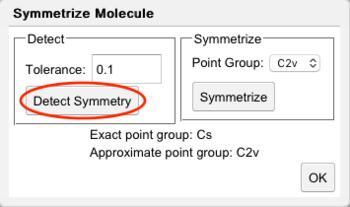Detect Symmetry Dialog

## Symmetrizing Molecules

An imported structure or the result of a calculation might be "nearly symmetric" while in reality such molecules may be expected to be fully symmetric. Also, quantum chemistry programs can take advantage of molecule symmetry to speed up calculations and reduce memory requirements. Thus, it is usually desirable to symmetrize nearly symmetric molecules prior to a calculation. WebMO will warn the user if one exits the Build Molecule page with a nearly symmetric molecule.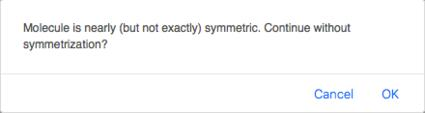Warning if Molecule is Nearly Symmetric

A nearly symmetric molecule is indicated by having its point group displayed in red with an asterisk as the Symmetrize icon (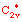). Clicking the icon will symmetrize the molecule by making the minimal necessary adjustments to the geometry. An exactly symmetric molecule is the indicated by having its point group displayed in red with an asterisk as the Symmetrize icon (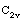).

Although clicking the Symmetrize icon usually has the desired effect with the program defaults, more control over the symmetrization process is available in the Calculate: Symmetry: Symmetrize Molecule... dialog box. Opening this dialog runs a Detect Symmetry calculation with the default tolerance and outputs the pre-symmetrized result to the java console as described above. Optionally, one can increase or decrease the tolerance and click Detect Symmetry to detect more or fewer potential symmetry elements and point groups. After choosing a point group or subgroup, clicking the Symmetrize button will symmetrize the molecule to that group.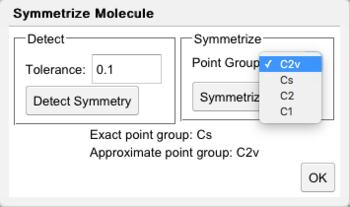Symmetrize Dialog

## Reference

WebMO uses the Largent-Polik-Schmidt algorithm for detecting potential symmetry elements, identifying point groups of nearly symmetry molecules, and symmetrizing nearly symmetric molecules: R.J. Largent, W.F. Polik, J.R. Schmidt, "Symmetrizer: Algorithmic Determination of Point Groups in Nearly Symmetric Molecules", J. Comput. Chem. 2012, 33, 1637-1642, DOI: 10.1002/jcc.22995.